# ECONOMICS EXPLORE APPLY by Ayers and Collinge Chapter

• Slides: 36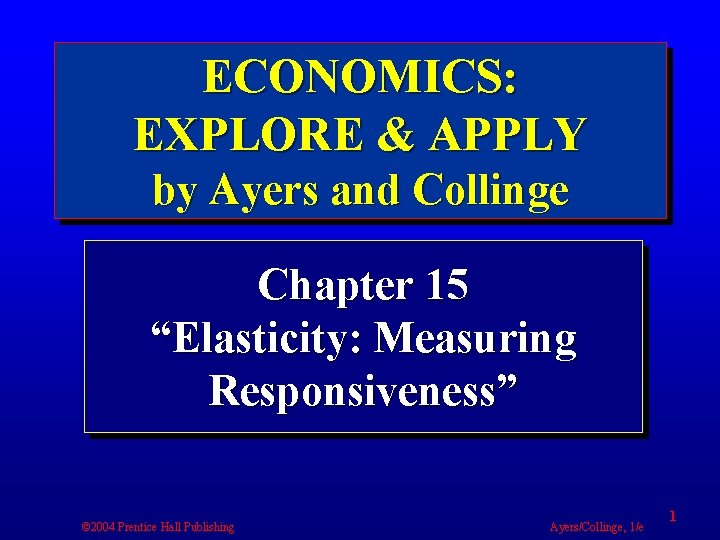ECONOMICS: EXPLORE & APPLY by Ayers and Collinge Chapter 15 “Elasticity: Measuring Responsiveness” © 2004 Prentice Hall Publishing Ayers/Collinge, 1/e 1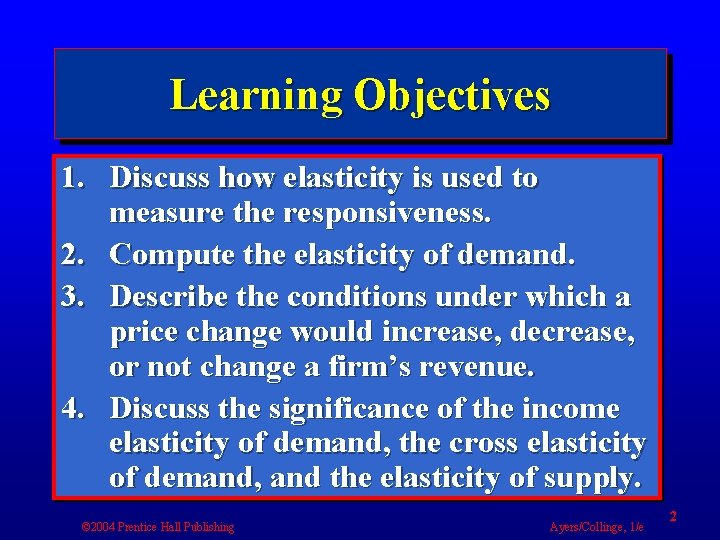Learning Objectives 1. Discuss how elasticity is used to measure the responsiveness. 2. Compute the elasticity of demand. 3. Describe the conditions under which a price change would increase, decrease, or not change a firm’s revenue. 4. Discuss the significance of the income elasticity of demand, the cross elasticity of demand, and the elasticity of supply. © 2004 Prentice Hall Publishing Ayers/Collinge, 1/e 2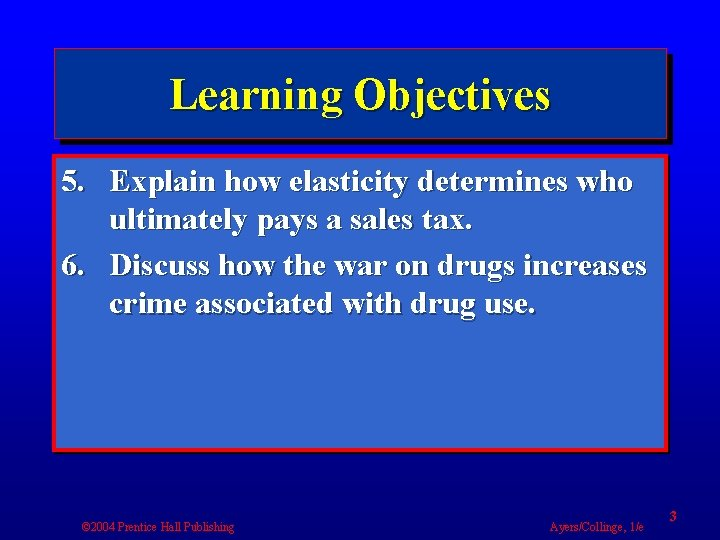Learning Objectives 5. Explain how elasticity determines who ultimately pays a sales tax. 6. Discuss how the war on drugs increases crime associated with drug use. © 2004 Prentice Hall Publishing Ayers/Collinge, 1/e 3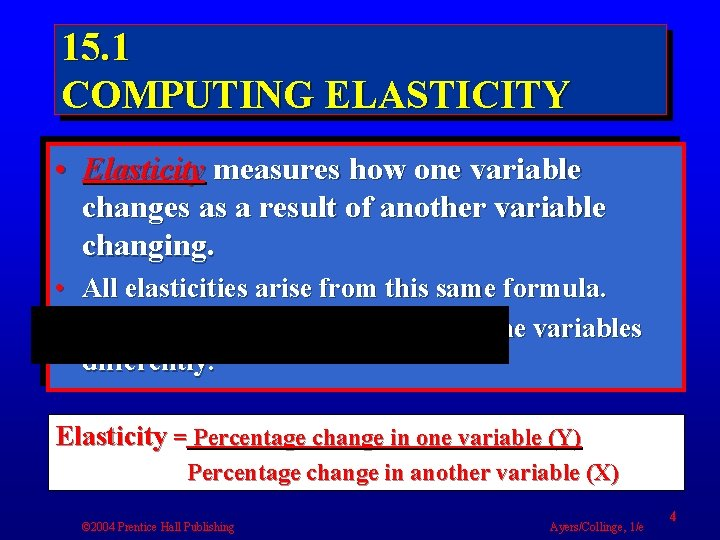15. 1 COMPUTING ELASTICITY • Elasticity measures how one variable changes as a result of another variable changing. • All elasticities arise from this same formula. • Different elasticities merely name the variables differently. Elasticity = Percentage change in one variable (Y) Percentage change in another variable (X) © 2004 Prentice Hall Publishing Ayers/Collinge, 1/e 4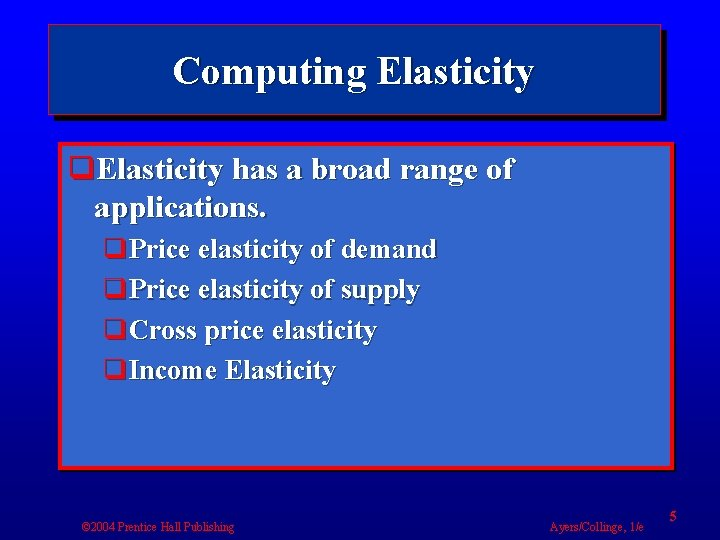Computing Elasticity q. Elasticity has a broad range of applications. q. Price elasticity of demand q. Price elasticity of supply q. Cross price elasticity q. Income Elasticity © 2004 Prentice Hall Publishing Ayers/Collinge, 1/e 5Midpoints Formula • The measure of percentage changes is provided by the midpoints formula. – The formula computes a percentage change as the change in the variable divided by an amount halfway between the starting and ending amount. Percentage change in Y= change in Y/Y midpoint Divided by Percentage change in X= change in X/X midpoint © 2004 Prentice Hall Publishing Ayers/Collinge, 1/e 6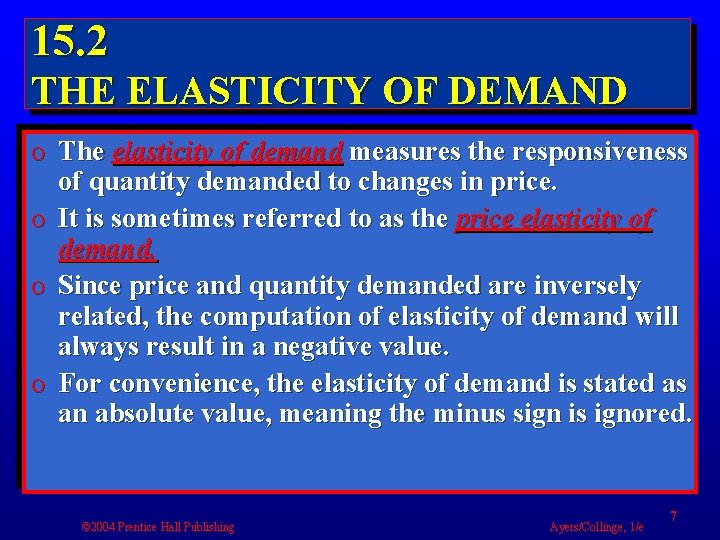15. 2 THE ELASTICITY OF DEMAND o The elasticity of demand measures the responsiveness of quantity demanded to changes in price. o It is sometimes referred to as the price elasticity of demand. o Since price and quantity demanded are inversely related, the computation of elasticity of demand will always result in a negative value. o For convenience, the elasticity of demand is stated as an absolute value, meaning the minus sign is ignored. © 2004 Prentice Hall Publishing Ayers/Collinge, 1/e 7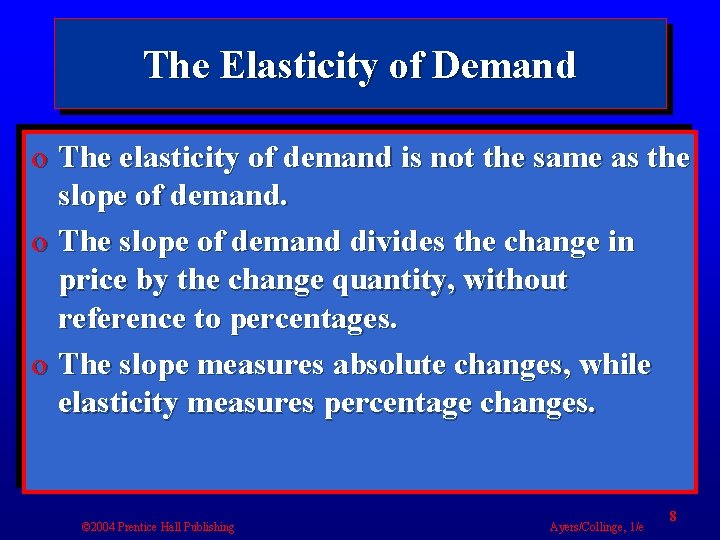The Elasticity of Demand o The elasticity of demand is not the same as the slope of demand. o The slope of demand divides the change in price by the change quantity, without reference to percentages. o The slope measures absolute changes, while elasticity measures percentage changes. © 2004 Prentice Hall Publishing Ayers/Collinge, 1/e 8Classifying the Elasticity of Demand § Inelastic demand has an elasticity coefficient whose value lies between 0 and 1. § Unit elastic demand has an elasticity coefficient whose value equals 1. § Elastic demand has an elasticity coefficient whose value is greater than 1. § Other things being equal, the more substitutes there are for a product, or the greater the fraction a product takes of a person’s budget to buy the product, the greater will be its elasticity of demand. © 2004 Prentice Hall Publishing Ayers/Collinge, 1/e 9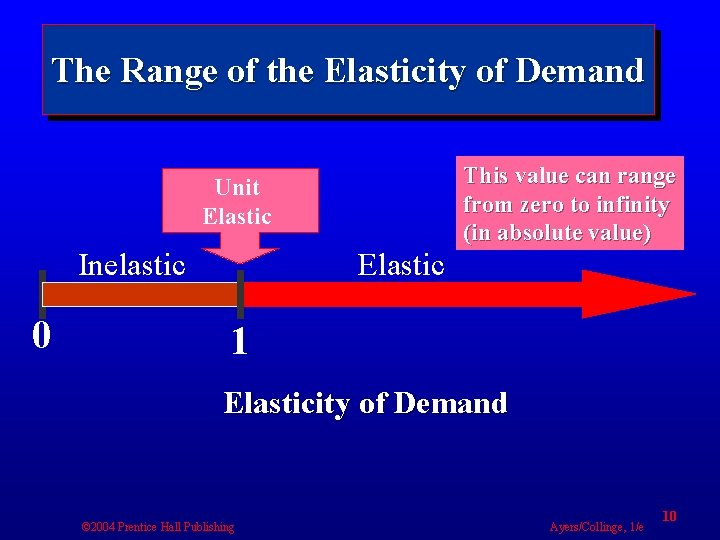The Range of the Elasticity of Demand Unit Elastic Inelastic 0 Elastic This value can range from zero to infinity (in absolute value) 1 Elasticity of Demand © 2004 Prentice Hall Publishing Ayers/Collinge, 1/e 10Estimates of Demand Elasticities Product Elasticity of Demand Beef 0. 35 Fish 0. 39 Public Transit 0. 40 Peak use electricity . 47 Physician services 0. 60 Poultry 0. 64 Fruit 0. 71 Peak use residential electricity 1. 5 Restaurant meals 2. 3 Kellogg’s Fruit Loops 2. 3 Cheerios 3. 6 Coke 3. 8 Mountain Dew 4. 4 Fresh Tomatoes 4. 6 © 2004 Prentice Hall Publishing Ayers/Collinge, 1/e 11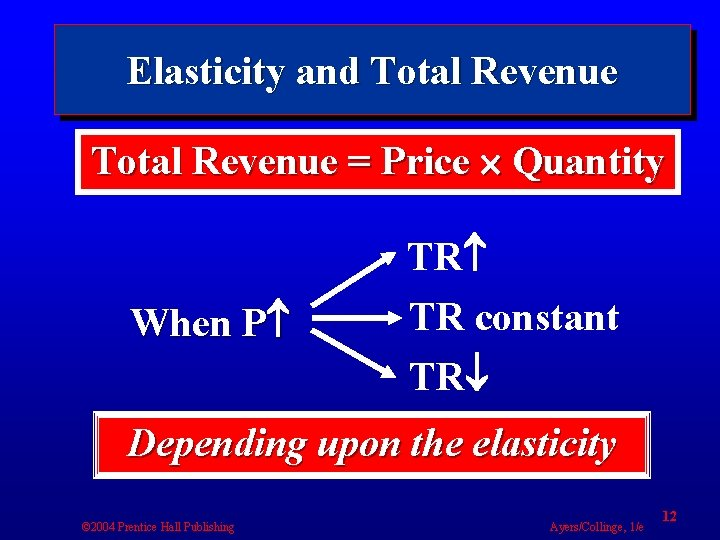Elasticity and Total Revenue = Price Quantity When P TR TR constant TR Depending upon the elasticity © 2004 Prentice Hall Publishing Ayers/Collinge, 1/e 12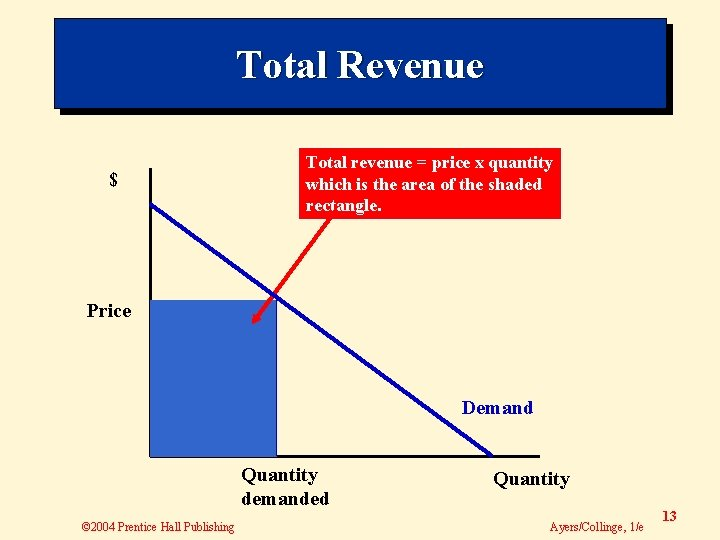Total Revenue \$ Total revenue = price x quantity which is the area of the shaded rectangle. Price Demand Quantity demanded © 2004 Prentice Hall Publishing Quantity Ayers/Collinge, 1/e 13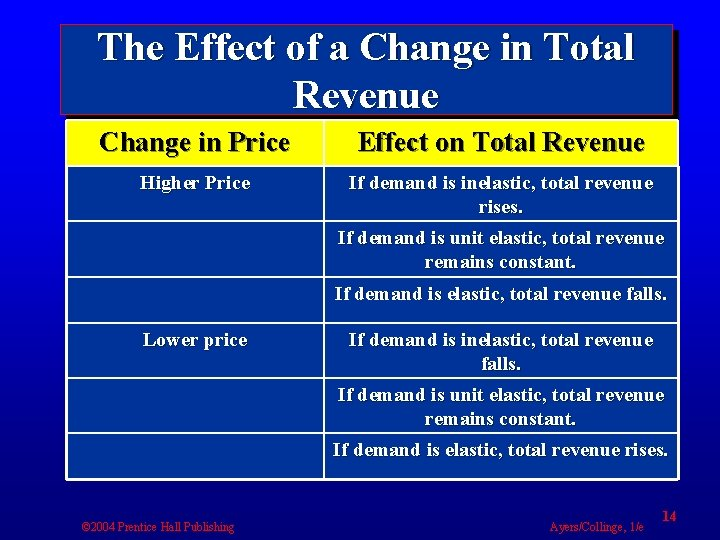The Effect of a Change in Total Revenue Change in Price Effect on Total Revenue Higher Price If demand is inelastic, total revenue rises. If demand is unit elastic, total revenue remains constant. If demand is elastic, total revenue falls. Lower price If demand is inelastic, total revenue falls. If demand is unit elastic, total revenue remains constant. If demand is elastic, total revenue rises. © 2004 Prentice Hall Publishing Ayers/Collinge, 1/e 14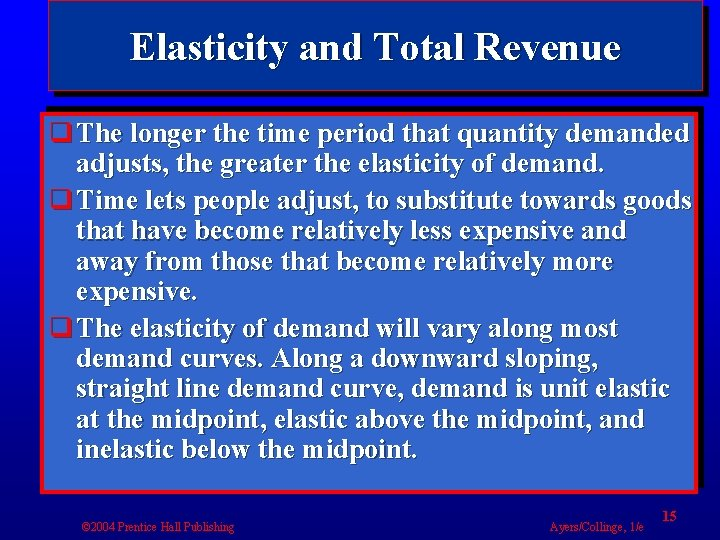Elasticity and Total Revenue q The longer the time period that quantity demanded adjusts, the greater the elasticity of demand. q Time lets people adjust, to substitute towards goods that have become relatively less expensive and away from those that become relatively more expensive. q The elasticity of demand will vary along most demand curves. Along a downward sloping, straight line demand curve, demand is unit elastic at the midpoint, elastic above the midpoint, and inelastic below the midpoint. © 2004 Prentice Hall Publishing Ayers/Collinge, 1/e 15Price (\$’s) Elasticity Varies along Linear Demand Curves Elastic Midpoint: Unit Elastic Inelastic Demand Quantity © 2004 Prentice Hall Publishing Ayers/Collinge, 1/e 16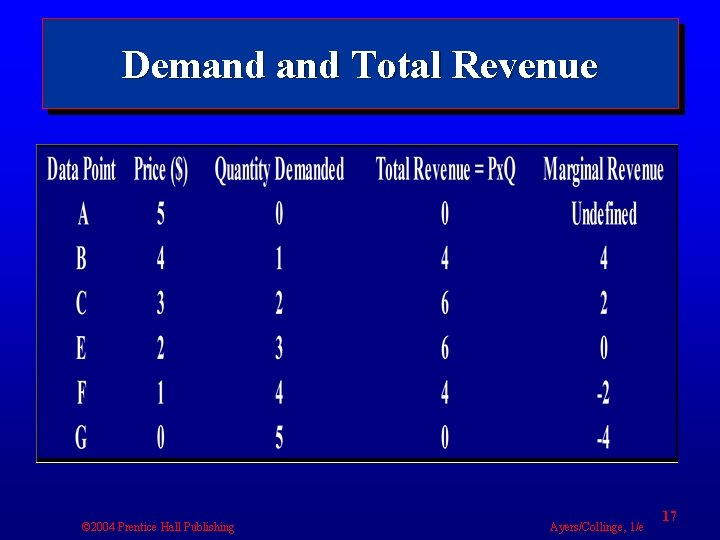Demand Total Revenue © 2004 Prentice Hall Publishing Ayers/Collinge, 1/e 17Computing the Elasticity of Demand Table 4 A-2 © 2004 Prentice Hall Publishing Ayers/Collinge, 1/e 18Dollars Demand, Elasticity, Total Revenue, and Marginal Revenue 5 A Total Revenue Elasticity =9 B 4 Elasticity =2. 33 C 3 Elasticity =1 D 2 Elasticity =. 43 E 1 Demand 0 1 © 2004 Prentice Hall Publishing 2 3 Elasticity =. 11 F Quantity 4 5 Marginal Revenue Ayers/Collinge, 1/e 19Dollars Demand, Elasticity, Total Revenue, and Marginal Revenue Total revenue is maximized when demand is unit elastic. Total Revenue Elastic Unit Elastic Each firm produces in the elastic range of its demand curve. Inelastic Demand Marginal Revenue © 2004 Prentice Hall Publishing Quantity Ayers/Collinge, 1/e 20Dollars The Extremes of Elasticity 6 Demand 3 1 Quantity 1. Perfectly Inelastic Elasticity = 0 © 2004 Prentice Hall Publishing 2 4 Quantity 2. Unit Elasticity =1 12 Quantity 3. Perfectly Elasticity = Ayers/Collinge, 1/e 2115. 3 INCOME AND CROSS ELASTICITIES OF DEMAND v The income elasticity of demand measures how demand responds to income. v It is computed by dividing the percentage change in quantity demanded, by the percentage in income. v A positive income elasticity indicates the good is a normal good. v A negative income elasticity of demand indicates the good is inferior. © 2004 Prentice Hall Publishing Ayers/Collinge, 1/e 22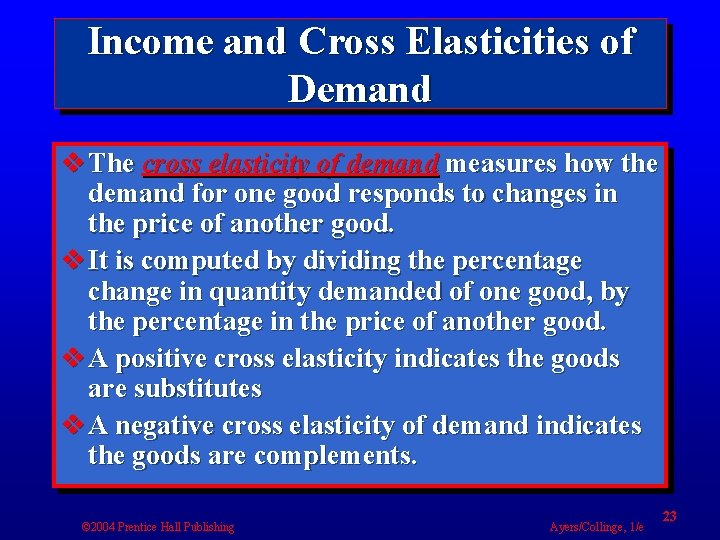Income and Cross Elasticities of Demand v The cross elasticity of demand measures how the demand for one good responds to changes in the price of another good. v It is computed by dividing the percentage change in quantity demanded of one good, by the percentage in the price of another good. v A positive cross elasticity indicates the goods are substitutes v A negative cross elasticity of demand indicates the goods are complements. © 2004 Prentice Hall Publishing Ayers/Collinge, 1/e 23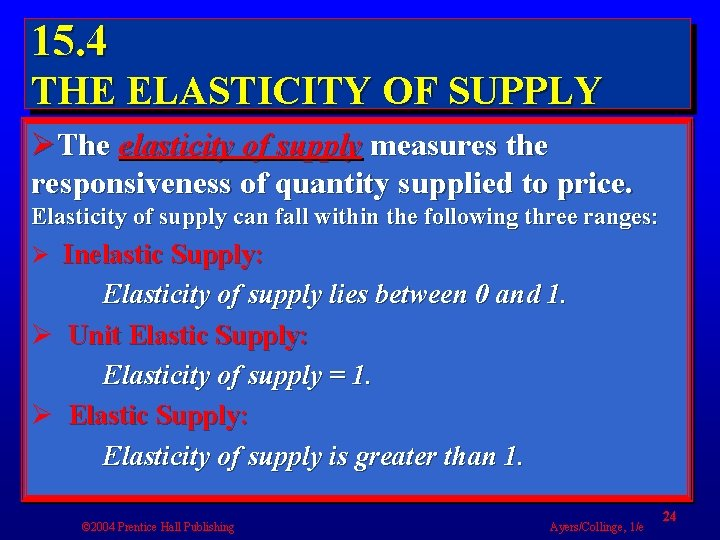15. 4 THE ELASTICITY OF SUPPLY ØThe elasticity of supply measures the responsiveness of quantity supplied to price. Elasticity of supply can fall within the following three ranges: Ø Inelastic Supply: Elasticity of supply lies between 0 and 1. Ø Unit Elastic Supply: Elasticity of supply = 1. Ø Elastic Supply: Elasticity of supply is greater than 1. © 2004 Prentice Hall Publishing Ayers/Collinge, 1/e 24Supply Dollars The Extremes of Supply Elasticity Supply Quantity 1. Perfectly Inelastic Elasticity = 0 2. Unit Elasticity =1 © 2004 Prentice Hall Publishing Quantity 3. Perfectly Elasticity = Ayers/Collinge, 1/e 2515. 5 TAX SHIFTING AND THE ELASTICITIES OF SUPPLY AND DEMAND Ø When a product is taxed, how much of that tax will the buyers pay, and how much of the tax will the sellers pay? The answer to that question of who ultimately shoulders the tax burden depends upon the demand supply elasticities of the product taxed. Ø Usually the tax burden is shared between producers and consumers. Ø Consumers pay more of the tax if demand is relatively less elastic than supply. Ø Producers pay more of the tax if demand is relatively more elastic than supply. © 2004 Prentice Hall Publishing Ayers/Collinge, 1/e 26Dollars Partial Tax Shifting #2 Consumers pay more. Supply plus Tax Supply Tax #2 sellers receive less. #1 The tax changes the market equilibrium to a higher price and a lower quantity. Demand Cigarettes © 2004 Prentice Hall Publishing Ayers/Collinge, 1/e 27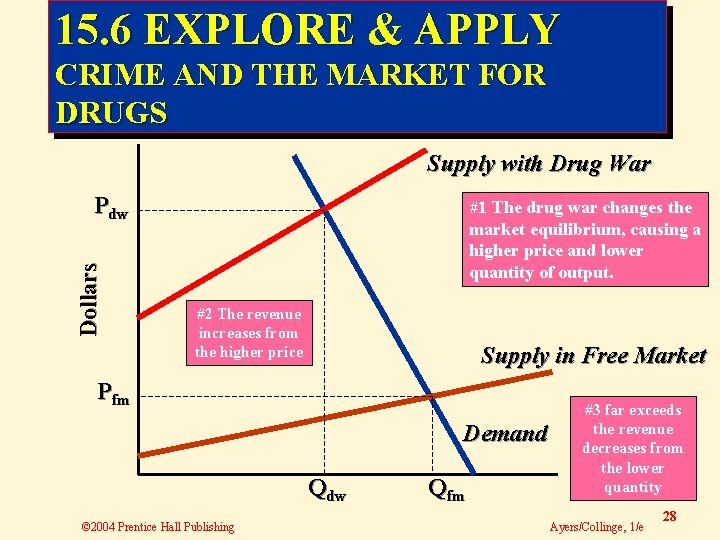15. 6 EXPLORE & APPLY CRIME AND THE MARKET FOR DRUGS Supply with Drug War Dollars Pdw #1 The drug war changes the market equilibrium, causing a higher price and lower quantity of output. #2 The revenue increases from the higher price Supply in Free Market Pfm Demand Qdw © 2004 Prentice Hall Publishing Qfm #3 far exceeds the revenue decreases from the lower quantity Ayers/Collinge, 1/e 28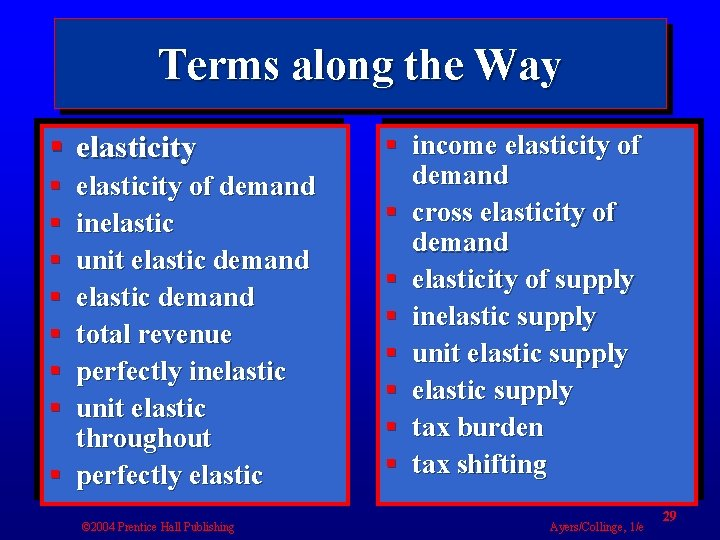Terms along the Way § elasticity § § § § elasticity of demand inelastic unit elastic demand total revenue perfectly inelastic unit elastic throughout § perfectly elastic © 2004 Prentice Hall Publishing § income elasticity of demand § cross elasticity of demand § elasticity of supply § inelastic supply § unit elastic supply § tax burden § tax shifting Ayers/Collinge, 1/e 29Test Yourself 1. The formula for all elasticities is the a. change in one variable divided by the change in another variable. b. change in one variable minus the change in another variable. c. percentage change in one variable divided by the percentage change in another variable. d. percentage change in one variable minus the percentage change in another variable. © 2004 Prentice Hall Publishing Ayers/Collinge, 1/e 30Test Yourself 2. Which of the following price elasticities of demand illustrates elastic demand? a. 0. 6 b. 0. 85 c. 1. 0 d. 1. 2 © 2004 Prentice Hall Publishing Ayers/Collinge, 1/e 31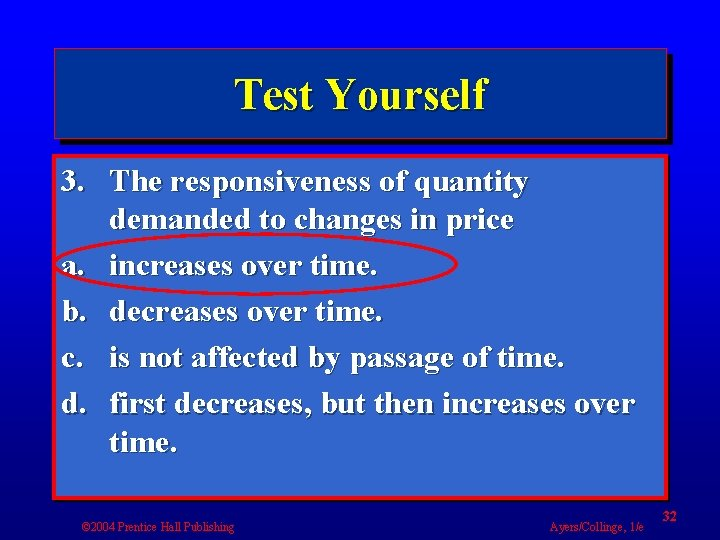Test Yourself 3. The responsiveness of quantity demanded to changes in price a. increases over time. b. decreases over time. c. is not affected by passage of time. d. first decreases, but then increases over time. © 2004 Prentice Hall Publishing Ayers/Collinge, 1/e 32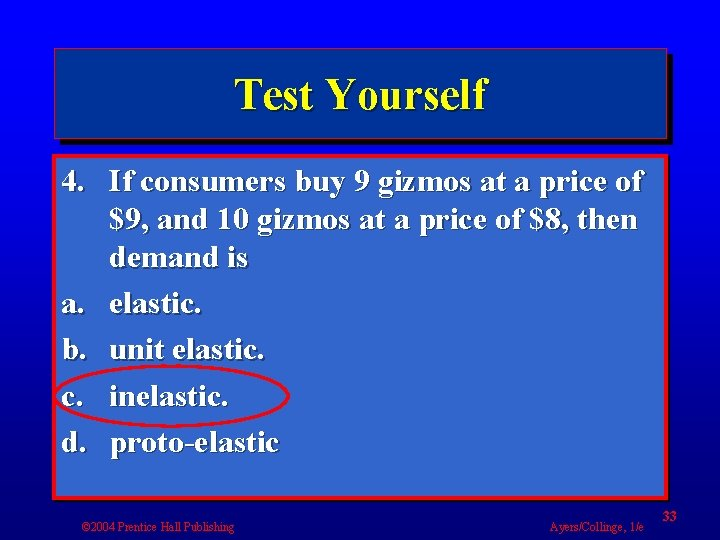Test Yourself 4. If consumers buy 9 gizmos at a price of \$9, and 10 gizmos at a price of \$8, then demand is a. elastic. b. unit elastic. c. inelastic. d. proto-elastic © 2004 Prentice Hall Publishing Ayers/Collinge, 1/e 33Test Yourself 5. An income elasticity of demand that is positive indicates a good that is a. normal. b. inferior. c. a substitute. d. a complement. © 2004 Prentice Hall Publishing Ayers/Collinge, 1/e 34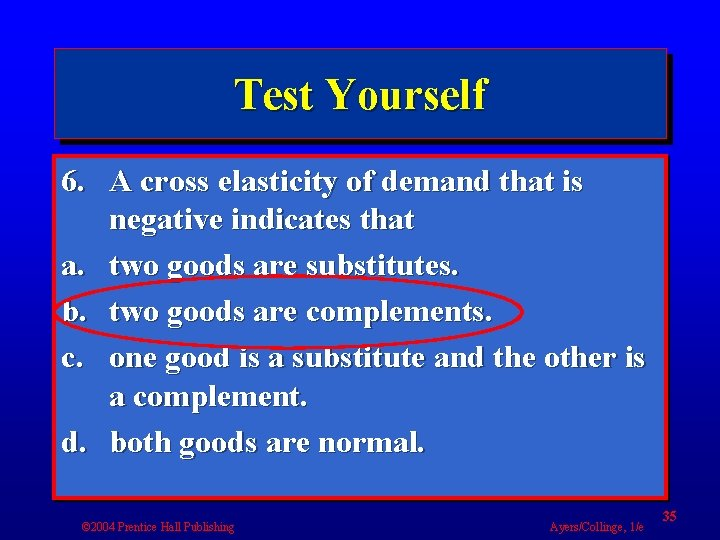Test Yourself 6. A cross elasticity of demand that is negative indicates that a. two goods are substitutes. b. two goods are complements. c. one good is a substitute and the other is a complement. d. both goods are normal. © 2004 Prentice Hall Publishing Ayers/Collinge, 1/e 35The End! Next Chapter 16 “Consumer Behavior" © 2004 Prentice Hall Publishing Ayers/Collinge, 1/e 36